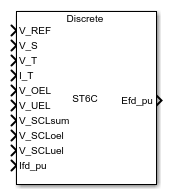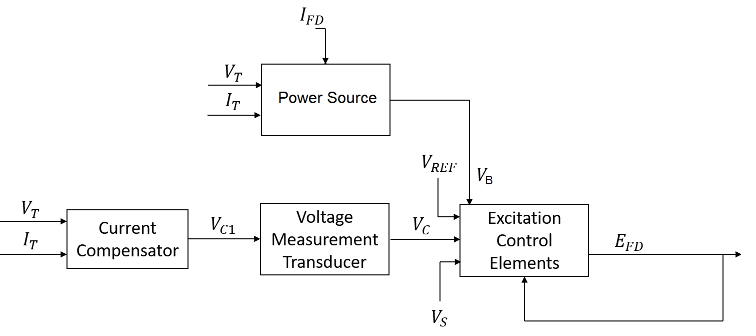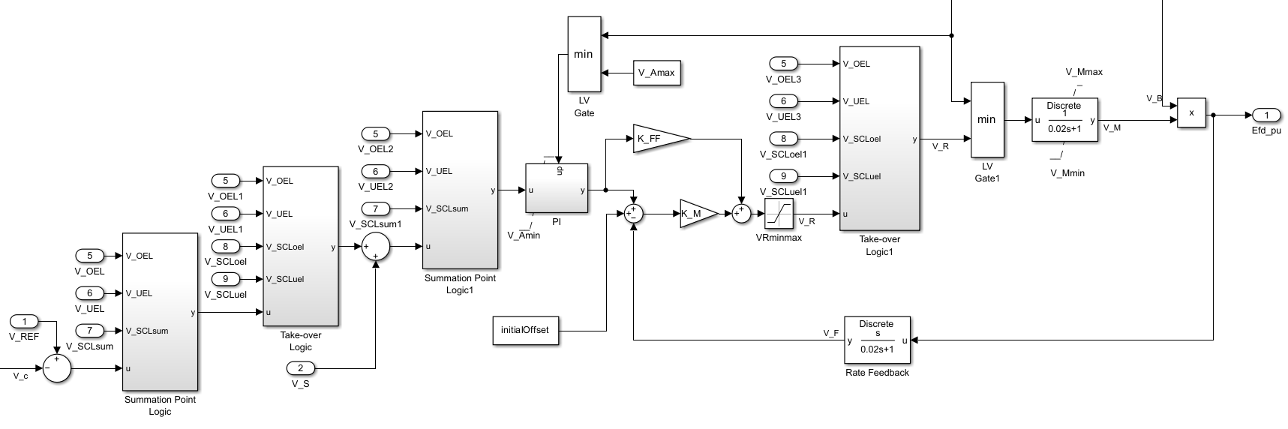# SM ST6C

Discrete-time or continuous-time synchronous machine ST6C static excitation system with automatic voltage regulator

Since R2023a

•Libraries:
Simscape / Electrical / Control / SM Control

## Description

The SM ST6C block implements a synchronous-machine-type ST6C static excitation system model in conformance with IEEE Std 421.5-2016 .

Use this block to model the control and regulation of the field voltage of a synchronous machine.

Switch between continuous and discrete implementations of the block by using the Sample time (-1 for inherited) parameter. To configure the integrator for continuous time, set the Sample time (-1 for inherited) parameter to `0`. To configure the integrator for discrete time, set the Sample time (-1 for inherited) parameter to a positive scalar. To inherit the sample time from an upstream block, set the Sample time (-1 for inherited) parameter to `-1`.

The SM ST6C block comprises four major components:

• The Current Compensator component modifies the measured terminal voltage as a function of the terminal current.

• The Voltage Measurement Transducer component simulates the dynamics of a terminal voltage transducer using a low-pass filter.

• The Excitation Control Elements component compares the voltage transducer output with a terminal voltage reference to produce a voltage error value. The component then passes this value through a voltage regulator to produce the field voltage.

• The Power Source component models the power source for the controlled rectifier when it is independent from the terminal voltage.

This diagram shows the structure of the ST6C excitation system model:In the diagram:

• VT and IT are the measured terminal voltage and current of the synchronous machine, respectively.

• VC1 is the current-compensated terminal voltage.

• VC is the filtered, current-compensated terminal voltage.

• VREF is the reference terminal voltage.

• VS is the power system stabilizer voltage.

• VB is the exciter field voltage.

• EFD and IFD are the field voltage and current, respectively.

### Current Compensator and Voltage Measurement Transducer

The block models the current compensator by using this equation:

`${V}_{C1}={V}_{T}+{I}_{T}\sqrt{{R}_{C}^{2}+{X}_{C}^{2}},$`

where:

• RC is the load compensation resistance.

• XC is the load compensation reactance.

The block implements the voltage measurement transducer as a Low-Pass Filter block with the time constant TR. Refer to the documentation for the Low-Pass Filter block for information about the exact discrete and continuous implementations.

### Excitation Control Elements

This diagram shows the structure of the excitation control elements:In the diagram:

• There are two Summation Point Logic subsystems. The subsystems model the summation point input locations for the overexcitation limiter (OEL), underexcitation limiter (UEL), stator current limiter (SCL), and power switch selector (V_S) voltages. For more information about using limiters with this block, see Field Current Limiters.

• There are two Take-over Logic subsystems. The subsystems model the take-over point input location for the OEL, UEL, SCL and PSS voltages. For more information about using limiters with this block, see Field Current Limiters.

• The PI subsystem models a PI controller that functions as a control structure for the automatic voltage regulator and allows the representation of an equipment retrofit with a modern digital controller. The minimum and maximum anti windup saturation limits for the block are VAmin and VAmax, respectively.

• An inner field voltage control loop is utilized to increase the dynamic response and it is composed of the gains KM and KFF. The minimum and maximum anti windup saturation limits for the block are VMmin and VMmax, respectively. If the field voltage regulator is not implemented, the corresponding parameters KG and KFF are set to 0.

• The Power source selector parameter controls the origin of the power source for the controlled rectifier. The subsystem then multiples the output of the rectifier bridge VM by the exciter field voltage VB. For more information about the logical switch for the power source of the controlled rectifier, see Power Source.

### Field Current Limiters

You can use different types of field current limiter to modify the output of the voltage regulator under unsafe operating conditions:

• Use an overexcitation limiter to prevent overheating of the field winding due to excessive field current demand.

• Use an underexcitation limiter to boost field excitation when it is too low, which risks desynchronization.

• Use a stator current limiter to prevent overheating of the stator windings due to excessive current.

Attach the output of any of these limiters at one of these points:

• Summation point — Use the limiter as part of the automatic voltage regulator (AVR) feedback loop.

• Take-over points — Override the usual behavior of the AVR.

If you are using the stator current limiter at the summation point, use the input VSCLsum. If you are using the stator current limiter at the take-over point, use the overexcitation input VSCLoel, and the underexcitation input VSCLuel.

### Power Source

You can use different power source representations for the controlled rectifier by setting the Power source selector parameter value. To derive the power source for the controlled rectifier from the terminal voltage, set the Power source selector parameter to ```Position A: power source derived from generator terminal voltage```. To specify that the power source is independent of the terminal voltage, set the Power source selector parameter to ```Position B: power source independent of generator terminal conditions```.

This diagram shows a model of the exciter power source utilizing a phasor combination of the terminal voltage VT and terminal current IT:## Ports

### Input

expand all

Voltage regulator reference set point, in per-unit representation, specified as a scalar.

Data Types: `single` | `double`

Input from the power system stabilizer, in per-unit representation, specified as a scalar.

Data Types: `single` | `double`

Terminal voltage magnitude, in per-unit representation, specified as a scalar.

Data Types: `single` | `double`

Terminal current magnitude, in per-unit representation, specified as a scalar.

Data Types: `single` | `double`

Input from the overexcitation limiter, in per-unit representation, specified as a scalar.

#### Dependencies

• To ignore the input from the overexcitation limiter, set Alternate OEL input locations (V_OEL) to `Unused`.

• To use the input from the overexcitation limiter at the summation point at voltage error, set Alternate OEL input locations (V_OEL) to ```Summation point at voltage error```.

• To use the input from the overexcitation limiter at the first take-over point, set Alternate OEL input locations (V_OEL) to ```Take-over at voltage error```.

• To use the input from the overexcitation limiter at the summation point at the AVR input, set Alternate OEL input locations (V_OEL) to ```Summation point at AVR input```.

• To use the input from the overexcitation limiter at the second take-over point, set Alternate OEL input locations (V_OEL) to ```Take-over at voltage regulator input```.

Data Types: `single` | `double`

Input from the underexcitation limiter, in per-unit representation, specified as a scalar.

#### Dependencies

• To ignore the input from the underexcitation limiter, set Alternate UEL input locations (V_UEL) to `Unused`.

• To use the input from the underexcitation limiter at the summation point at voltage error, set Alternate UEL input locations (V_UEL) to ```Summation point at voltage error```.

• To use the input from the underexcitation limiter at the first take-over point, set Alternate UEL input locations (V_UEL) to ```Take-over at voltage error```.

• To use the input from the underexcitation limiter at the summation point at the AVR input, set Alternate UEL input locations (V_UEL) to ```Summation point at AVR input```.

• To use the input from the underexcitation limiter at the second take-over point, set Alternate UEL input locations (V_UEL) to ```Take-over at voltage regulator input```.

Data Types: `single` | `double`

Input from the stator current limiter when using the summation point, in per-unit representation, specified as a scalar.

#### Dependencies

• To ignore the input from the stator current limiter, set Alternate SCL input locations (V_SCL) to `Unused`.

• To use the input from the stator current limiter at the summation point, set Alternate SCL input locations to any of the `Summation point` options.

Data Types: `single` | `double`

Input from the stator current limiter that prevents field overexcitation when using the take-over point, in per-unit representation, specified as a scalar.

#### Dependencies

• To ignore the input from the stator current limiter, set Alternate SCL input locations (V_SCL) to `Unused`.

• To use the input from the stator current limiter at the take-over point, set Alternate SCL input locations (V_SCL) to any of the `Take-over` options.

Data Types: `single` | `double`

Input from the stator current limiter that prevents field underexcitation when using the take-over point, in per-unit representation, specified as a scalar.

#### Dependencies

• To ignore the input from the stator current limiter, set Alternate SCL input locations (V_SCL) to `Unused`.

• To use the input from the stator current limiter at the take-over point, set Alternate SCL input locations (V_SCL) to any of the `Take-over` options.

Data Types: `single` | `double`

Measured per-unit field current of the synchronous machine, specified as a scalar.

Data Types: `single` | `double`

### Output

expand all

Per-unit field voltage to apply to the field circuit of the synchronous machine, returned as a scalar.

Data Types: `single` | `double`

## Parameters

expand all

### General

Initial per-unit voltage to apply to the field circuit of the synchronous machine.

Initial per-unit voltage to apply to the terminal.

#### Dependencies

To enable this parameter, in the Exciter section, set Power source selector to ```Position A: power source derived from generator terminal voltage```.

Initial per-unit current to apply to the terminal.

#### Dependencies

To enable this parameter, in the Exciter section, set Power source selector to ```Position A: power source derived from generator terminal voltage```.

Time between consecutive block executions. During execution, the block produces outputs and, if appropriate, updates its internal state. For more information, see What Is Sample Time? and Specify Sample Time.

For inherited discrete-time operation, set this parameter to `-1`. For discrete-time operation, set this parameter to a positive integer. For continuous-time operation, set this parameter to `0`.

If this block is in a masked subsystem or a variant subsystem that supports switching between continuous operation and discrete operation, promote this parameter to ensure correct switching between the continuous and discrete implementations of the block. For more information, see Promote Block Parameters on a Mask.

### Pre-Control

Resistance used in the current compensation system. Set this parameter and Reactance component of load compensation, X_C (pu) to `0` to disable current compensation.

Reactance used in the current compensation system. Set this parameter and Resistive component of load compensation, R_C (pu) to `0` to disable current compensation.

Equivalent time constant for the voltage transducer filtering.

### Control

Proportional gain of the voltage regulator PI control block.

Integral gain of the voltage regulator PI control block.

Per-unit pre-control gain constant of the inner-loop field voltage regulator. To disable the inner-loop field voltage regulator, set this parameter and the Feedback gain constant of the inner loop field voltage regulator, K_G parameter to `0`.

Per-unit forward gain of the inner-loop field voltage regulator.

Per-unit feedback gain constant of the inner-loop field voltage regulator. To disable the inner-loop field voltage regulator, set this parameter and the Pre-control gain constant of the inner loop field voltage regulator, K_FF parameter to `0`.

Feedback time constant of the inner-loop field voltage regulator.

Maximum per-unit output of the voltage regulator.

Minimum per-unit output of the voltage regulator.

Maximum per-unit regulator output.

Minimum per-unit regulator output.

Maximum per-unit rectifier output.

Minimum per-unit rectifier output.

Equivalent time constant of the firing control of the thyristor bridge, in seconds.

Location of the overexcitation limiter input, specified as one of these options:

• ```Summation point at voltage error```V_OEL is an input of the Summation Point Logic subsystem.

• `Take-over at voltage error`V_OEL is an input of the Take-over Logic subsystem.

• `Summation point at AVR input`V_OEL is an input of the Summation Point Logic1 subsystem.

• ```Take-over at voltage regulator output```V_OEL is an input of the Take-over Logic 1 subsystem.

Location of the underexcitation limiter input, specified as one of these options:

• ```Summation point at voltage error```V_UEL is an input of the Summation Point Logic subsystem.

• `Take-over at voltage error`V_UEL is an input of the Take-over Logic subsystem.

• `Summation point at AVR input`V_UEL is an input of the Summation Point Logic1 subsystem.

• ```Take-over at voltage regulator output```V_UEL is an input of the Take-over Logic 1 subsystem.

Stator current limiter input location, specified as one of these options:

• ```Summation point at voltage error```V_SCL is an input of the Summation Point Logic subsystem.

• `Take-over at voltage error`V_SCL is an input of the Take-over Logic subsystem.

• `Summation point at AVR input`V_SCL is an input of the Summation Point Logic1 subsystem.

• ```Take-over at voltage regulator output```V_SCL is an input of the Take-over Logic 1 subsystem.

### Exciter

Maximum per-unit exciter current limiter gain.

Per-unit exciter current limit reference.

Per-unit rectifier loading factor. This value is proportional to the commutating reactance.

Per-unit potential circuit gain coefficient.

Per-unit potential circuit current gain coefficient.

#### Dependencies

To enable this parameter, set Power source selector SW1 to ```Position A: power source derived from generator terminal voltage```.

Per-unit reactance associated with the potential source.

#### Dependencies

To enable this parameter, set Power source selector to ```Position A: power source derived from generator terminal voltage```.

Potential circuit phase angle, in degrees.

#### Dependencies

To enable this parameter, set Power source selector to ```Position A: power source derived from generator terminal voltage```.

Maximum per-unit available field voltage for the exciter.

Position of the power source selector.

 IEEE Std 421.5-2016 (Revision of IEEE Std 421.5-2005). "IEEE Recommended Practice for Excitation System Models for Power System Stability Studies." Piscataway, NJ: IEEE, 2016.